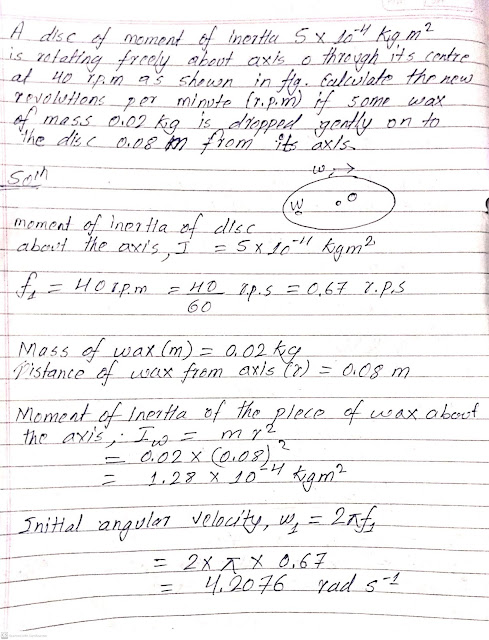# A disc of moment of inertia 5 x 10^-4 kg m^2 is rotating freely about axis O through its centre at 40 rpm as shown in figure.Calculate the new revolution per minute(r.p.m) if some wax of mass 0.02kg is dropped gently on to the disc 0.08m from its axis.

(Physics Class 12 | Rotational Dynamics)A disc of moment of inertia 5 x 10^-4 kg m^2 is rotating freely about axis O through its centre at 40 rpm as shown in figure.Calculate the new revolution per minute(r.p.m) if some wax of mass 0.02kg is dropped gently on to the disc 0.08m from its axis.